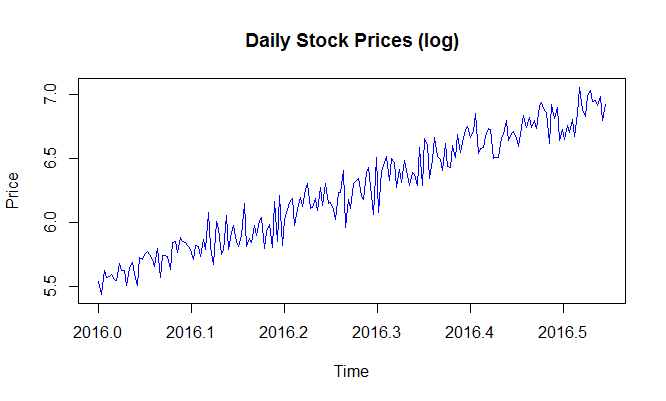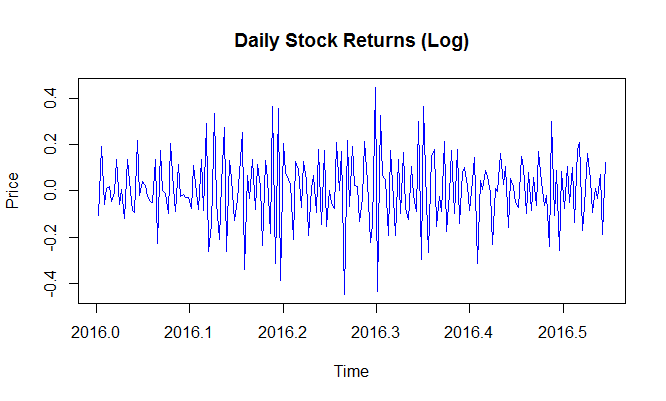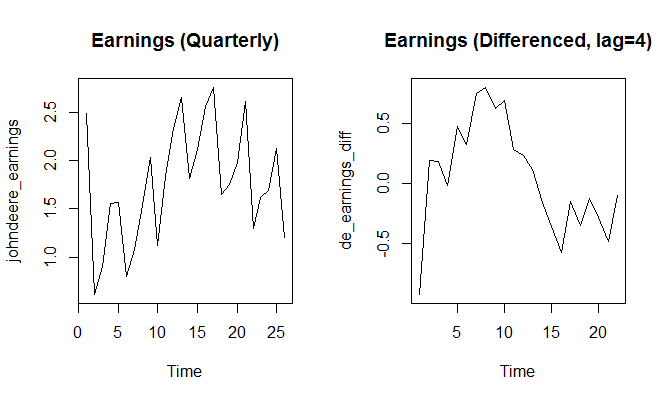# Differencing and Log Transformation

### Removing Variability Using Logarithmic Transformation

Since the data shows changing variance over time, the first thing we will do is stabilize the variance by applying log transformation using the log() function. The resulting series will be a linear time series.

> sp_linear<-log(sp_ts)
> plot.ts(sp_linear, main="Daily Stock Prices (log)", ylab="Price", col=4)### Removing Linear Trend

We will now perform the first difference transformation [z(t) - z(t-1)] to our series to remove the linear trend.

> sp_linear_diff <- diff(sp_linear)
> plot.ts(sp_linear_diff, main="Daily Stock Prices (log)", ylab="Price", col=4)
>### Removing Seasonal Differencing

Let's take another example to understand how we can use the diff() function to remove seasonal differencing from data. We will use the John Deer's Quarterly earnings data we used earlier as it exhibits seasonality.

> par(mfrow = c(1,2))
> de_earnings_diff <- diff(johndeere_earnings,lag=4)
> plot.ts(johndeere_earnings, main="Earnings (Quarterly)")
> plot.ts(de_earnings_diff, main="Earnings (Differenced, lag=4)")


The chart on the left shows the original earnings. The chart on the right shows the difference in earnings with a lag of 4.Get our Data Science for Finance Bundle for just $29$51.